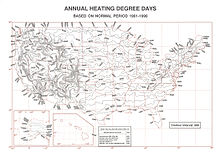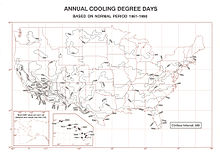# Heating degree day

﻿
Heating degree dayUnited States Heating Degree Day map, 1961-1990United States Cooling Degree Day map, 1961-1990

Heating degree day (HDD) is a measurement designed to reflect the demand for energy needed to heat a home or business. It is derived from measurements of outside air temperature. The heating requirements for a given structure at a specific location are considered to be directly proportional to the number of HDD at that location. A similar measurement, cooling degree day (CDD), reflects the amount of energy used to cool a home or business.

Heating degree days are defined relative to a base temperature - the outside temperature above which a building needs no heating. The most appropriate base temperature for any particular building depends on the temperature that the building is heated to, and the nature of the building (including the heat-generating occupants and equipment within it).

For calculations relating to any particular building, HDD should be selected with the most appropriate base temperature for that building. However, for historical reasons HDD are often made available with base temperatures of 18.3°C (65°F), or 15.5°C (60°F) - base temperatures that are approximately appropriate for a good proportion of buildings.

There are a number of ways in which HDD can be calculated: the more detailed a record of temperature data, the more accurate the HDD that can be calculated. HDD are often calculated using simple approximation methods that use daily temperature readings instead of more detailed temperature records such as half-hourly readings. One popular approximation method is to take the average temperature on any given day, and subtract it from the base temperature. If the value is less than or equal to zero, that day has zero HDD. But if the value is positive, that number represents the number of HDD on that day. This method works satisfactorily if the outside air temperature does not exceed the base temperature. In climates where this is likely to occur from time to time, there are refinements to the simple calculation which allow some 'credit' for the period of the day when the air is warm enough for heating to be unnecessary. This more accurate algorithm enables results to be computed in temperate climates (maritime as well as continental) throughout the year (not just during a defined heating season) and on a weekly as well as monthly basis.

HDD can be added over periods of time to provide a rough estimate of seasonal heating requirements. In the course of a heating season, for example, the number of HDD for New York City is 5,050 whereas that for Barrow, Alaska is 19,990. Thus, one can say that, for a given home of similar structure and insulation, around four times the energy would be required to heat the home in Barrow than in New York. Likewise, a similar home in Los Angeles, California, whose heating degree days for the heating season is 2020, would require around two fifths the energy required to heat the house in New York City.

Example: For a typical New York City winter day with High = 40°F and Low = 30°F, the Average Temperature is likely to be around 35°F. For such a day we can approximate the HDD as (65 - 35) = 30. A month of thirty similar days might accumulate HDD = 900. A year (including summer average temperatures above 70°F) might accumulate an annual HDD = 5000.

## Example of use

HDD provides a simple metric for quantifying the amount of heating that buildings in a particular location need over a certain period (e.g. a particular month or year). In conjunction with the average U-value for a building they provide a means of roughly estimating the amount of energy required to heat the building over that period.

One HDD means that the temperature conditions outside the building were equivalent to being below a defined threshold comfort temperature inside the building by one degree for one day. Thus heat has to be provided inside the building to maintain thermal comfort. Say we are given the number of heating degree days D in one year and we wish to calculate the energy required by a building. We know that heat needs to be provided at the rate at which it is being lost to the environment. This can be calculated as the sum of the heat losses per degree of each element of the buildings' thermal envelope (such as windows, walls, and roof) or as the average U-value of the building multiplied by the area of the thermal envelope of the building, or quoted directly for the whole building. This gives the buildings' specific heat loss rate Pspecific, generally given in Watts per Kelvin (W/K). Total energy in kWh is then given by:

Q = Pspecific×24×D/1000 [kWh]

Note that as total energy consumption is in kWh and heating degree days are [no. days×degrees] we must convert W/K into kWh per degree per day by dividing by 1000 (to convert W to kW), and multiplying by 24 hours in a day (1 kW = 1kWh per hour). Since one degree temperature difference in Celsius and Kelvin scale are the same, they get cancelled and no conversion is required.

## Problems

Calculations using HDD have several problems. Heat requirements are not linear with temperature, and heavily insulated buildings have a lower "balance point". The amount of heating and cooling required depends on several factors besides outdoor temperature: How well insulated a particular building is, the amount of solar radiation reaching the interior of a house, the number of electrical appliances running (e.g. computers raise their surrounding temperature) the amount of wind outside, and individuals' opinions about what constitutes a comfortable indoor temperature. Another important factor is the amount of relative humidity indoors; this is important in determining how comfortable an individual will be. Other variables such as wind speed, precipitation, cloud cover, heat index, and snow cover can also alter a building's thermal response.

Another problem with HDD is that care needs to be taken if they are to be used to compare climates internationally, because of the different baseline temperatures used as standard in different countries and the use of the Fahrenheit scale in the US and the Celsius scale almost everywhere else. This is further compounded by the use of different approximation methods in different countries.

## Conversion

To convert °F HDD to °C HDD: °C HDD = (5/9) x (°F HDD)

To convert °C HDD to °F HDD: °F HDD = (9/5) x (°C HDD)

Note that, because HDD are relative to a base temperature (as opposed to being relative to zero), it is incorrect to add or subtract 32 when converting degree days from Celsius to Fahrenheit or vice versa.

Wikimedia Foundation. 2010.

### Look at other dictionaries:

• heating degree-day — a degree day below the standard temperature of 65°F or 19°C, used in estimating fuel consumption. Cf. cooling degree day, growing degree day. * * * …   Universalium

• heating degree-day — a degree day below the standard temperature of 65°F or 19°C, used in estimating fuel consumption. Cf. cooling degree day, growing degree day …   Useful english dictionary

• heating degree day — (HDD)    see degree day …   Dictionary of units of measurement

• Heating Degree Day(s) — (HDD)   The number of degrees per day that the daily average temperature (the mean of the maximum and minimum recorded temperatures) is below a base temperature, usually 65 degrees Fahrenheit, unless otherwise specified; used to determine indoor… …   Energy terms

• Heating degree day —   A unit that measure the space heating needs during a given period of time.   California Energy Comission. Dictionary of Energy Terms …   Energy terms

• heating degree day (HDD) — A day in which the average daily temperature is less than 65 degrees Fahrenheit, and therefore likely to be a day in which people turn on their heat. A heating degree day is assigned a value that represents the number of degrees that days average …   Financial and business terms

• Heating Degree Day - HDD — The number of degrees that a day s average temperature is below 65o Fahrenheit (18o Celsius), the temperature below which buildings need to be heated. The price of weather derivatives traded in the winter is based on an index made up of monthly… …   Investment dictionary

• Degree day — A degree day is a measure of heating or cooling. Totalized degree days from an appropriate starting date are used to plan the planting of crops and management of pests and pest control timing. Weekly or monthly degree day figures may also be used …   Wikipedia

• Degree Day —   A unit for measuring the extent that the outdoor daily average temperature (the mean of the maximum and minimum daily dry bulb temperatures) falls below (in the case of heating, see Heating Degree Day), or falls above (in the case of cooling,… …   Energy terms

• Heating Degree Days — (HDD)   A measure of how cold a location is over a period of time relative to a base temperature, most commonly specified as 65 degrees Fahrenheit. The measure is computed for each day by subtracting the average of the day s high and low… …   Energy terms